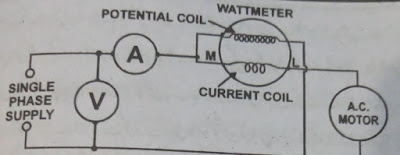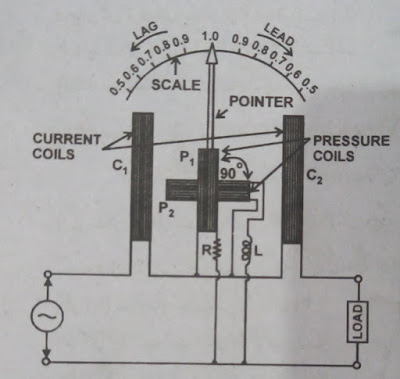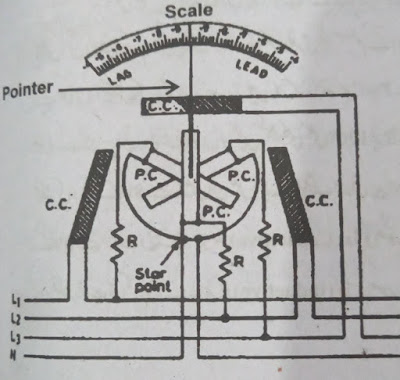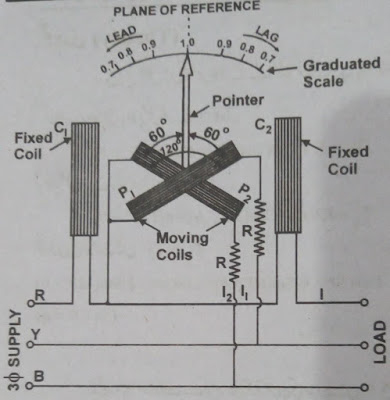# Power Factor Meter-Power Factor Meter Formula Circuit- Power Factor Meter Types Construction and Working Principle-2 and 3 Phases Meters

In this article, we are going to tell you about Power Factor Meters. Also, the types, structure, principles, and working methods of the power factor meters are explained in detail. Also, power factor meter diagrams are included.

# Power Factor Meter

The power factor is the cosine of the angle (phase angle) ɸ between the current passing through an AC circuit and the voltage found parallel to the load.

Power factor meters are meters that measure the power factor of a circuit and display it directly on its scale. These are called power factor meters. In industries, if a consumer's power factor is less than the standard power factor, then that consumer has to pay a penalty. (WAPDA Standard Power Factor is currently 0.9 leaking) Power Factor Meters are installed in industries to check or monitor individual load, any group of loads, or the total load power factor of the industry. To avoid the penalty that has to be paid in case of a low power factor.

Or

The ratio between the actual power (KW) and the external power (KVA) of the load obtained in an AC circuit is called the power factor. It is denoted by cos ɸ.

## Power Factor Formula

Power Factor = True Power (KW)/Apparent Power (KVA)

Power Factor Cos ɸ = W/VA

## Circuit of Power Factor

Wattmeter shows real power. The product of the ampere meter and voltmeter readings is called the apparent power. The power factor of a single fair load can be determined by connecting these three meters to the circuit according to the shape. In this case, dividing the wattmeter reading by the voltmeter and ampere meter reading gives the power factor of the load. In practice, this method of determining the power factor is not easy. Therefore, meters have been developed which can directly measure the power factor of AC load.Power Factor Meter

## Types of Power Factor Meters

Power factor meters are mainly divided into two classes.

### 1. Types of power factor meters in terms of supply

• Single Phase Power Factor Meters
• Three-Phase Power Factor Meters
• Power factor meters for three-phase balanced load
• Power factor meters for three phases unbalance load

### 2. Types of power factor meters in terms of structure

• Moving Coil Power Factor Meters
• Moving Iron Power Factor Meters

### Single Phase Dynamometer Type P.F Meters

A device that measures the power factor in a single-phase AC circuit, under the influence of the mechanical force found between the two current-carrying conductors. The single-phase dynamometer type is called a power factor. This power factor meter is also called a coming power coil or power factor meter. It is also called a quadrature coil power factor meter. It is also called crossed coil power factor meter.

### Working Principle

The working principle of dynamometer type moving coil power factor meter (and to a considerable extent also the same) is the same. Which is a dynamometer-type moving coil wattmeter. The torque coming into the meter's moving system depends on the interaction of the magnetic field of the current coils and the pressure coils. The motion of the moving system (clockwise or counter-clockwise) depends on the nature of the load current, whether the load current is inductive or resistive.Single Phase Meters

### Theory

Suppose the power factor of the load with which the power factor meter is fitted, the moving system (i.e. both moving coils P1 and P2) for this value of the power factor is balanced by rotating ɸ angle from its first position.

TP1 = KViL Cos ɸ * Sin Ѳ

TP2 = KViL Cos(90 - ɸ) * Sin(90 + Ѳ)

TP1 = TP2

Or          Cos ɸ*Sin Ѳ = Cos(90 - ɸ)*Sin(90 – Ѳ)

Or          Tan Ѳ = Tan ɸ

Or          Ѳ = ɸ

### Three Phase Dynamometer Type P.F meter (for Balance Load)

Three Phase Dynamometer Type Power Factor Meter is used to determine the power factor of three-phase balanced load.

### Construction

The figure shows the structure and connection of the three-phase dynamometer type power factor for a balanced load. It has C1 and C2 double wire loops of thick wire. These have been added to the series in a series of three-round supply red phases.

The line current passes through both of them. Between these two fixed coils, two pressure coils P1 and P2 are connected at 120° and fitted on a pivoted spindle. Therefore, these two move together. In the P1 series, high resistance R is added to connect between Y (i.e. Yellow) phase and R (i.e. Red) phase.Three Phase Meter

### Working Method

To generate torque in a single-phase supply, artificial arrangements have to be made to obtain a phase shift.

But in this instrument, since the moving coils are connected to two phases of the three-phase system (which differ from each other by 120° electrical degrees depending on the difference), there is a need for artificial phase shifting. Does not fall since both the coils are also mechanically connected to 120. Therefore, in the case of balance load (depending on the value of power factor of the load), rotating torque is generated in them. Which moves the pointer from the unit position according to the phase angle of the circuit. In the case of the capacitive load, this movement will be on the left. But in practice, the leading power factor is very low. In the case of inductive load, the pointer will move to the right.

### Theory

T1 = KVRY iCos(30 + ɸ) Sin(60 + Ѳ)

T2 = KVRB iCos(30 - ɸ) Sin(120 + Ѳ)

Cos(30 - ɸ) Sin(60 + Ѳ) = Cos(30 - ɸ) Sin(120 + Ѳ)

### Tree Phase Dynamometer Types P.F Meter (for Unbalance Load)

A three-wire three-phase system is usually balanced. Because this supply is given to three-phase machines. The three-phase four-wire system, on the other hand, is generally unbalanced. Because of this single-phase supply is also obtained. Which is obtained from a single-phase and neutral. Thus the system becomes unbalanced due to different loads on the three phases.

### Working Principle

In the above sequence, two magnetic fields are formed, one due to pressure coils and the other due to current coils. Both magnetic fields are automatically rotating without any additional arrangement. Because the three phases of three-phase supply are at 120 (electrical) degrees.Three Phase Meter

If the load is purely resistive (in this case the power factor is unit), then the magnetic field of the coils and the magnetic field of the current coils will be in phase with each other. And both will rotate at synchronous speed. Therefore, no mechanical torque will be generated on the moving system (pressure coils). That way she will stay still. And the measuring needle unit will stand on the power factor. If there is some inductance or capacitance in the load then both the magnetic fields are not in phase. Due to this, the torque will act on the moving system and it will tell the corresponding power factor by moving left or right depending on the power factor of the load.